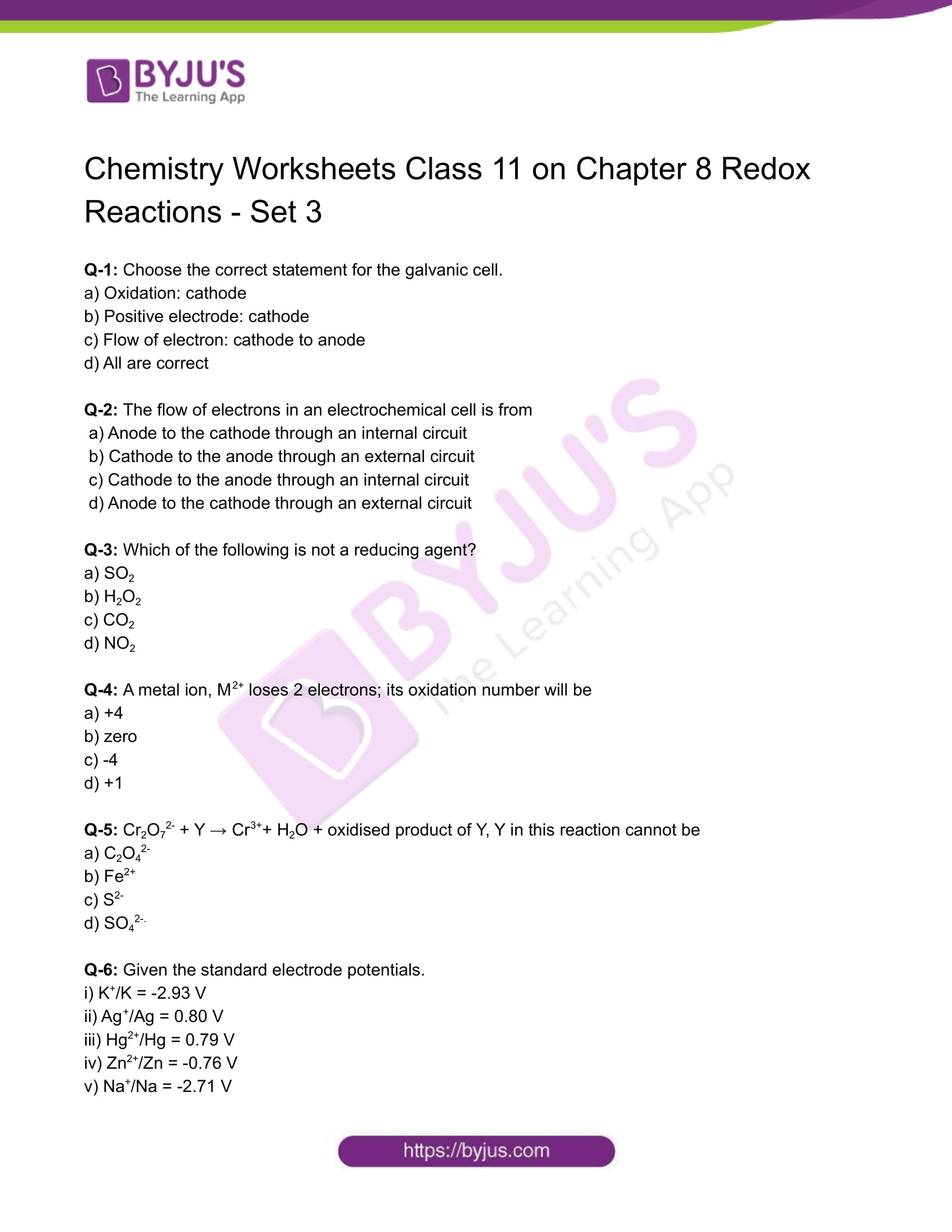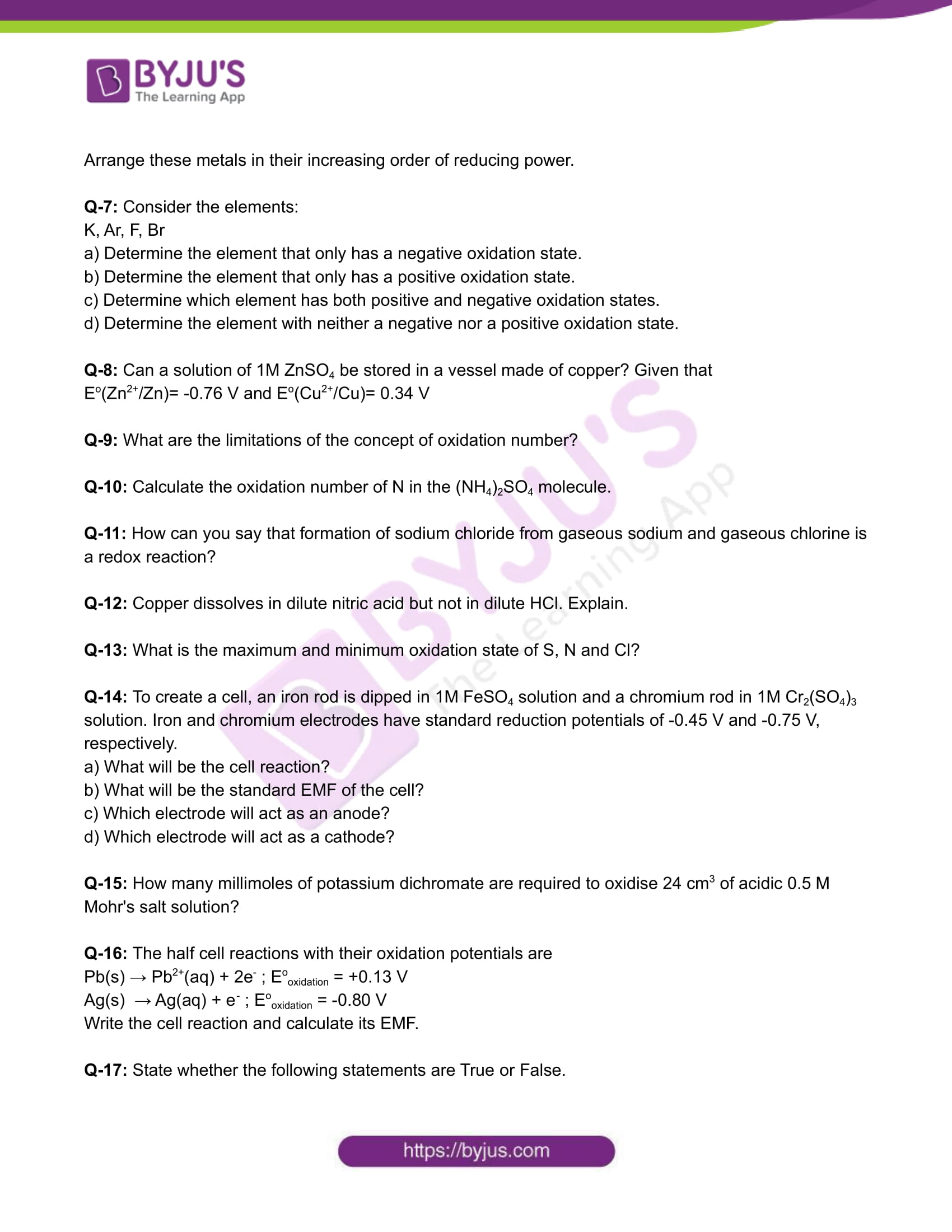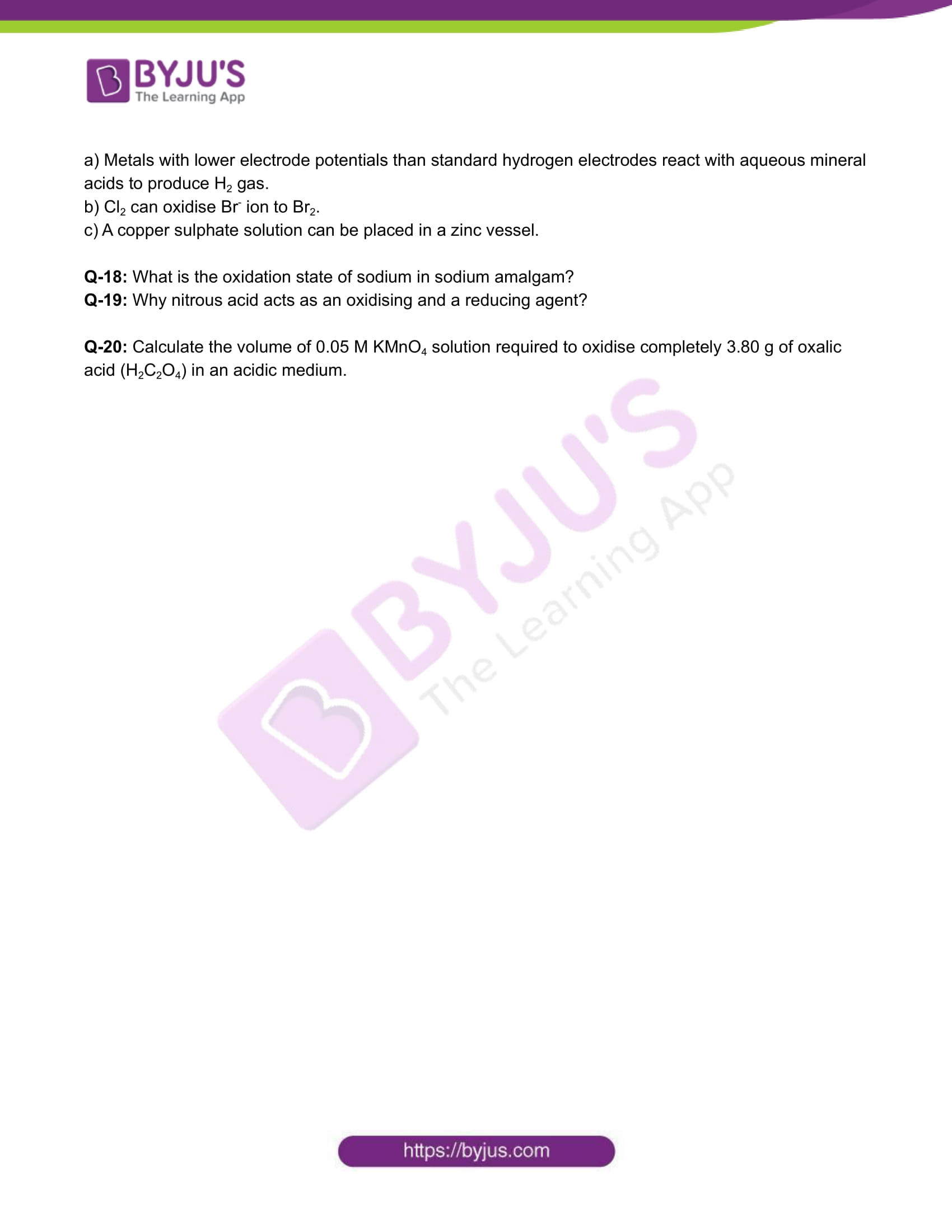Oxidation and Reduction Reactions – Basic Introduction
Oxidation and Reduction Reactions – Basic Introduction

The oxidation states of the reactants change during redox reactions, which are oxidation-reduction chemical processes. Reduction-oxidation is referred to as redox. All redox reactions can be divided into two categories: reduction processes and oxidation processes. There are always concurrent oxidation and reduction reactions in redox or oxidation-reduction reactions. The substance being reduced in a chemical reaction is known as the reducing agent, and the substance being oxidised is known as the oxidising agent.

Download Class 11 Chemistry Worksheet on Chapter 8 Redox Reactions Set 3 PDF.## CBSE Class 11 Chemistry Chapter 8 Redox Reactions– Set 3

Q-1: Choose the correct statement for the galvanic cell.

a) Oxidation: cathode

b) Positive electrode: cathode

c) Flow of electron: cathode to anode

d) All are correct

Q-2: The flow of electrons in an electrochemical cell is from

a) Anode to the cathode through an internal circuit

b) Cathode to the anode through an external circuit

c) Cathode to the anode through an internal circuit

d) Anode to the cathode through an external circuit

Q-3: Which of the following is not a reducing agent?

a) SO2

b) H2O2

c) CO2

d) NO2

Q-4: A metal ion, M2+ loses 2 electrons; its oxidation number will be

a) +4

b) zero

c) -4

d) +1

Q-5: Cr2O72- + Y → Cr3++ H2O + oxidised product of Y, Y in this reaction cannot be

a) C2O42-

b) Fe2+

c) S2-

d) SO42-.

Q-6: Given the standard electrode potentials.

i) K+/K = -2.93 V

ii) Ag+/Ag = 0.80 V

iii) Hg2+/Hg = 0.79 V

iv) Zn2+/Zn = -0.76 V

v) Na+/Na = -2.71 V

Arrange these metals in their increasing order of reducing power.

Q-7: Consider the elements:

K, Ar, F, Br

a) Determine the element that only has a negative oxidation state.

b) Determine the element that only has a positive oxidation state.

c) Determine which element has both positive and negative oxidation states.

d) Determine the element with neither a negative nor a positive oxidation state.

Q-8: Can a solution of 1M ZnSO4 be stored in a vessel made of copper? Given that

Eo(Zn2+/Zn)= -0.76 V and Eo(Cu2+/Cu)= 0.34 V

Q-9: What are the limitations of the concept of oxidation number?

Q-10: Calculate the oxidation number of N in the (NH4)2SO4 molecule.

Q-11: How can you say that formation of sodium chloride from gaseous sodium and gaseous chlorine is a redox reaction?

Q-12: Copper dissolves in dilute nitric acid but not in dilute HCl. Explain.

Q-13: What is the maximum and minimum oxidation state of S, N and Cl?

Q-14: To create a cell, an iron rod is dipped in 1M FeSO4 solution and a chromium rod in 1M Cr2(SO4)3 solution. Iron and chromium electrodes have standard reduction potentials of -0.45 V and -0.75 V, respectively.

a) What will be the cell reaction?

b) What will be the standard EMF of the cell?

c) Which electrode will act as an anode?

d) Which electrode will act as a cathode?

Q-15: How many millimoles of potassium dichromate are required to oxidise 24 cm3 of acidic 0.5 M Mohr’s salt solution?

Q-16: The half cell reactions with their oxidation potentials are

Pb(s) → Pb2+(aq) + 2e– ; Eooxidation = +0.13 V

Ag(s) → Ag(aq) + e– ; Eooxidation = -0.80 V

Write the cell reaction and calculate its EMF.

Q-17: State whether the following statements are True or False.

a) Metals with lower electrode potentials than standard hydrogen electrodes react with aqueous mineral acids to produce H2 gas.

b) Cl2 can oxidise Br– ion to Br2.

c) A copper sulphate solution can be placed in a zinc vessel.

Q-18: What is the oxidation state of sodium in sodium amalgam?

Q-19: Why nitrous acid acts as an oxidising and a reducing agent?

Q-20: Calculate the volume of 0.05 M KMnO4 solution required to oxidise completely 3.80 g of oxalic acid (H2C2O4) in an acidic medium.

Download the PDF to access answers to the Chemistry Worksheet for Class 11 Chemistry Chapter 8 Redox Reactions Set – 3.

You are watching: Class 11 Chemistry Worksheet on Chapter 8 Redox Reactions. Info created by Bút Chì Xanh selection and synthesis along with other related topics.